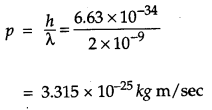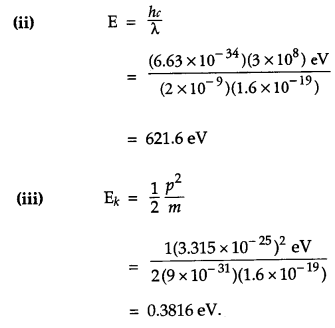## BSE Previous Year Question Papers Class 12 Physics 2011 Delhi

### CBSE Previous Year Question Papers Class 12 Physics 2011 Delhi Set-I

Question 1.
A point charge is placed at point O as shown in the figure. Is the potential difference VA-VB positive, negative or zero, if Q is
(i) positive
(ii) negative ?If Q is positively charged, VA-VB = positive.
If Q is negatively charged, VA-VB = negative.

Question 2.
A plane electromagnetic wave travels in vacuum along Z-direction. What can you say about the direction of electric and magnetic field vector ? 
In electromagnetic wave, the electric field vector $$\overrightarrow{\mathrm{E}}$$ and magnetic field vector $$\overrightarrow{\mathrm{B}}$$ show their variations perpendicular to the direction of propagation of wave as well as perpendicular to each other. As the electromagnetic wave is travelling along Z-direction, hence $$\overrightarrow{\mathrm{E}}$$ and $$\overrightarrow{\mathrm{B}}$$ show their variation in XY-plane.

Question 3.
A resistance R is connected across a cell of emf 8 and internal resistance r. A potentiometer now measures the potential difference between the terminals of the cell as V. Write the expression for r in terms of ∈, V and R. 
$$r=\left(\frac{\varepsilon}{V}-1\right) \times R$$

Question 4.
The permeability of magnetic material is 0.9983. Name the type of magnetic materials it represents. 
Diamagnetic material.

Question 5.
Show graphically, the variation of the de- Broglie wavelength (λ,) with the potential (V) through which an electron is accelerated from rest.Question 6.
In a transistor, doping level in base is increased slightly. How will it affect
(i) collector current and
(ii) base current ? 

Question 7.
Define the term ‘watt less current’. 
Current flowing in a circuit without any net dissipation of power is called watt less current.

Question 8.
When monochromatic light travels from one medium to another its wavelength changes but frequency remains the same. Explain. 
Atoms (of the second medium) oscillate with the same (incident light) frequency and in turn, emit light of the same frequency.

Question 9.
Two Uniformly large parallel thin plates having charge densities +σ and -σ are kept in the X-Z plane at a distance ‘d’ apart. Sketch an equipotential surface due to electric field between the plates. If a particle of mass m and charge ‘-q’ remains stationary between the plates, what is the magnitude and direction of this field ? 
OR
Two small identical electrical dipoles AB and CD, each of dipole moment ‘p’ are kept at an angle of 120° as shown in the figure. What is the resultant dipole moment of this combination ? if this system is subjected to electric field E directed along +X direction, what will be the magnitude and direction of the torque acting on this ?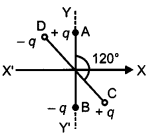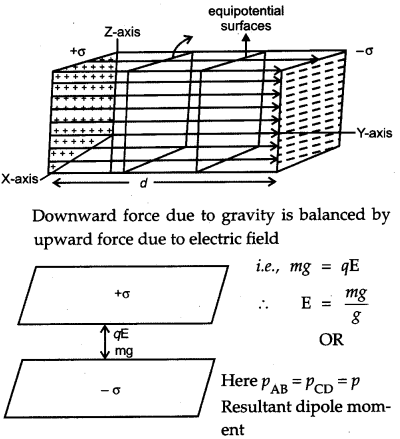Question 10..
A magnetic needle free to rotate in a vertical plane parallel to the magnetic meridian has its north tip down at 60° with the horizontal. The horizontal component of the earth’s magnetic field at the place is known to be 0.4 G. Determine the magnitude of the earth’s magnetic field at the place. 
Given, angle of dip, δ= 60°Question 11.
Figure shows two identical capacitors, C1 and C2, each of 1 µF capacitance connected to a battery of 6 V. Initially switch ‘S’ is closed. After sometime ‘S’ is left open and dielectric slabs of dielectric constant K = 3 are inserted to fill completely the space between the plates of the two capacitors. How will the
(i) charge and
(ii) potential difference between the plates of the capacitors be affected after the slabs are inserted ?Question 12.
Two convex lenses of same focal length but of aperture A1 and A2 (A2 < A1), are used as the objective lenses in two astronomical telescopes having identical eyepieces. What is the ratio of their resolving power ? Which telescope will you prefer and why ? Give reason. 
Resolving power of a telescope is proportional to its aperture, and is given by a relation,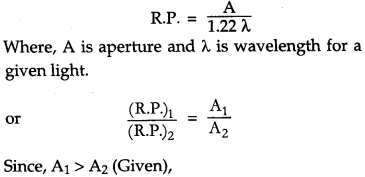Therefore, the telescope with objective of aperture A1 should be preferred for viewing as this would:
(i) give a better resolution.
(ii) have a higher light gathering power of telescope.

Question 13.
Draw the output waveform at X, using the given inputs A and B for the logic circuit shown below. Also, identify the logic operation performed by this circuit.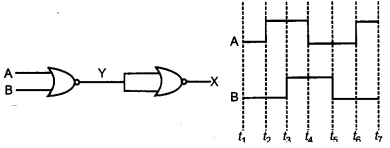Question 14.
Name the semiconductor device that can be used to regulate an unregulated dc power supply. With the help of I-V characteristics of this device, explain its working principle. 
Zener diode is a specially designed diode which is operated in reverse breakdown region continuously without any damage. When zener diode is operated in the reverse break down region, the voltage across it remains practically constant (VZ) for a large change in reverse current. Therefore, for any increase/ decrease of the input voltage, there is a increase/ decrease of the voltage drop across series resistance (Rs) without any change in the voltage across zener diode.Question 15.
How are infrared waves produced ? Why are these referred to as ‘heat wave’ ? Write their one important use. 
Infrared rays are produced by hot bodies or due to vibration of atoms and molecules of the bodies. Infrared waves are called heat waves as they cause heating effect. Infrared waves are used to maintain earth’s warmth, in physical therapy, remote switches etc.

Question 16.
Draw the transfer characteristic curve of a base biased transistor in CE configuration. Explain clearly how the active region of the V0 versus V; curve in a transistor is used as an amplifier. 

Question 17.
(a) Define modulation index.
(b) Why is the amplitude of modulating signal kept less than the amplitude of carrier wave ? 

Question 18.
A current is induced in coil C1 due to the motion of current carrying coil C2.
(a) Write any two ways by which a large deflection can be obtained in the galvanometer G.
(b) Suggest an alternative device to demonstrate the induced current in place of a galvanometer.(a) Any two ways to obtain large deflection in G:

• Moving C2 faster towards Q.
• Insertion of soft iron core in C1.

(b) Alternative device that can be used in place of galvanometer is LED.

Question 19.
Define the terms
(i) drift velocity,
(ii) relaxation time.
A conductor of length L is connected to a dc source of emf ∈. If this conductor is replaced by another conductor of same material and same area of cross-section but of length 3L, how will the drift velocity change ? 
(i) Drift velocity: The average velocity with which the free electrons drift towards positive terminal under the influence of an external electric field is called drift velocity.
(ii) Relaxation time : Average time interval between two successive collisions of an electron with the positive ions / atoms of the conductor. Drift velocity is given by,
$$\begin{array}{l}{v_{d}=\frac{\sigma \tau}{m} \frac{\mathrm{E}}{\mathrm{L}}} \\ {v_{d} \propto \frac{1}{\mathrm{L}}}\end{array}$$
The drift velocity is inversely proportional to L.
If length L of conductor is made 3 times, drift velocity will become one-third of its initial value.
$$v_{d}^{\prime}=\frac{v_{d}}{3}$$

Question 20.
Using Gauss’s law obtain the expression for the electric field due to a uniformly charged thin spherical shell of radius R at a point outside the shell. Draw a graph showing the variation of electric field with r, for r > R and r < R. 
Electric field at point P at a distance ‘r’ outside the spherical shell.
According to Gauss’ law,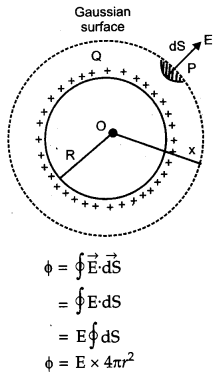For r < R, there is no strength of electric field inside a charged spherical shell.
For r > R, electric field outside a spherical shell is same as if the whole charge Q is concentrated at the center.

Question 21.
An electron and a photon each have a wavelength 1.00 nm. Find
(a) their momenta,
(b) the energy of the photon, and
(c) the kinetic energy of electron.Question 22.
Draw a schematic diagram showing the
(i) ground wave
(ii) sky wave and
(iii) space wave propagation modes for em waves.
Write the frequency range for each of the following: 
(ii) Television
(iii) Satellite communication.

Question 23.
Describe Young’s double slit experiment to produce interference pattern due to a monochromatic source of light. Deduce the expression for the fringe width. 
OR
Use Huygens’s principle to verify the laws of refraction.
S is a narrow slit (of width about 1 mm) illuminated by a monochromatic source of light. At a suitable distance (= 10 mm) from S, two slits S1 and S2 are placed parallel to S. When a screen is placed at a large distance (about 2 m) from the slit S1 and S2, alternate dark and bright bands appear on the screen. These are the interference bands or fringes. The band disappear when either slit is covered.Explanation : According to Huygens’s principle, the monochromatic source of light illuminating the slit S sends out spherical wave front.

The two waves of same amplitude and same frequency superimpose on each other. Dark fringes appear on the screen when the crest of one wall falls on the trough of other and they neutralize the effect of each other. Bright fringes appear on the screen when the crest of one wave coincides with the crest of other and they reinforce each other.

Expression for the fringe width:
Let d = distance between slits S1 and S2,
D = distance of screen from two slits, and
x = distance between the central maxima
‘O’ and observation point P.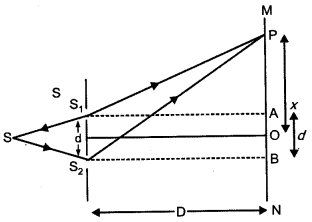Light waves spread out from S and fall on both S1 and S2. The spherical waves emanating from S1 and S2 will produce interference fringes on the screen MN.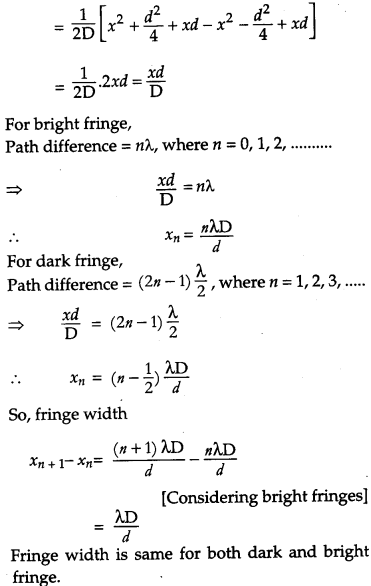OR
Wave front : The continuous locus of all the particles of a medium, which are vibrating in the same phase is called wave front.
Laws of refraction: Let PP’ represent the surface separating medium 1 and medium 2 as shown in fig.which is Snell’s law of refraction of light (first law).
Second law : Incident wave front, refracted wave front, normal’s all lie in the same plane.

Question 24.
(a) Describe briefly, with the help of suitable diagram, how the transverse nature of light can be demonstrated by the phenomenon of polarization.
(b) When unpolarized light passes from air to a transparent medium, under what condition does the reflected light get polarized ? 
(a) When a polaroid P1 is rotated in the path of an unpolarized light, there is no change in transmitted intensity. The light transmitted through polaroid P1 is made to pass through polaroid P2. On rotating polaroid P2 in path of light transmitted from PA we notice a change in intensity of transmitted light. This shows the light transmitted from P1 is polarized. Since light can be polarized, it has transverse nature.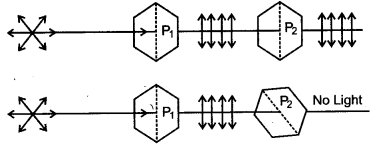(b) Whenever unpolarized light is incident from air to a transparent medium at an angle of incidence equal to polarizing angle, the reflected light gets fully polarized.
According to Brewster’s law,
$$n=\tan i_{p}$$ …….. (i)
where ip in the polarizing angle and n is refractive index of the transparent material.
By Snell’s law, we have .
or \begin{aligned} n &=\frac{\sin i_{p}}{\sin r} \\ \text { or } & \tan i_{p}=\frac{\sin i_{p}}{\sin r} \end{aligned}  [From (i)]
\begin{aligned} \therefore \quad & \cos i_{p}=\sin r \\ & \cos i_{p}=\cos \left(90^{\circ}-r\right) \\ \therefore & i_{p}+r=90^{\circ} \end{aligned}
Thus, when the sum of polarizing angle and reflected angle is 90°, the reflected light is fully polarized.

Question 25.
The energy levels of a hypothetical atom are shown below. Which of the shown transitions will result in the emission of a photon of wavelength 275 nm ? Which of these transitions correspond to emission of radiation of
(i) maximum and
(ii) minimum wavelength ?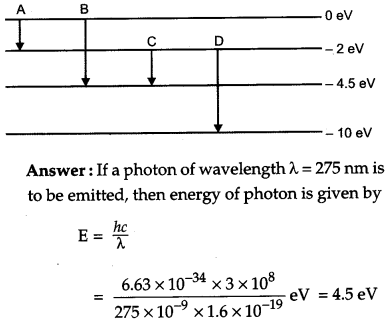Hence transition B would result in the emission of a photon of wavelength 275 nm.
(i) Transition A corresponds to maximum wavelength.
(ii) Transition D corresponds to minimum wavelength.

Question 26.
State the law of radioactive decay.
Plot a graph showing the number (N) of undecayed nuclei as a function of time (t) for a given radioactive sample having half life T1/2.
Depict in the plot the number of undecayed nuclei at
(i) t = 3 T1/2 and
(ii) t = 5 T1/2. 
The number of nuclei undergoing decay per unit time, at any instant t, is proportional to the total number of nuclei in the sample at that instant.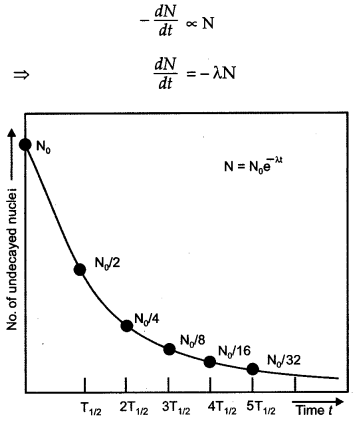From graph, number of undecayed nuclei at
(i) 3 T1/2 is No/8.
(ii) 5 T1/2 is N0/32.

Question 27.
In the circuit shown, R1 = 4 Ω, R2 = R3 = 15 Ω, R4 = 30 Ω and E = 10 V. Calculate the equivalent resistance of the circuit and the current in each resistor.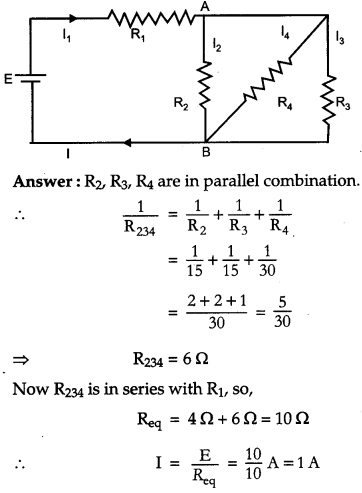Question 28.
State Biot-Savart’s law, giving the mathematical expression for it.
Use this law to derive the expression for the magnetic field due to a circular coil carrying current at a point along its axis.
How does a circular loop carrying current behave as a magnet ? 
OR
With the help of a labelled diagram, state the underlying principle of a cyclotron. Explain clearly how it works to accelerate the charged particles. Show that cyclotron frequency is independent of energy of the particle. Is there an upper limit on the energy acquired by the particle ? Give reason.
Biot-Savart’s law states that the magnitude of magnetic field dB due to current element is directly proportional to the current I, the element length | dl | and inversely proportional to the square of the distance r of the field point. Its direction is perpendicular to the plane containing $$\overrightarrow{d l}$$ and $$\overrightarrow{r}$$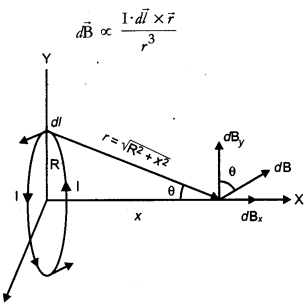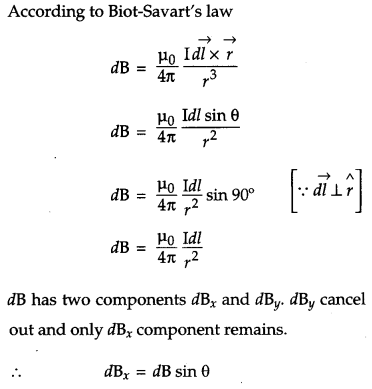Explanation : A magnetic needle placed at the center or axis of a circular coil shows deflection. This implies that a circular coil behaves as a magnet.
OR
Principle: Cyclotron works on the principle that a charged particle moving normal to a magnetic field experiences Lorentz magnetic force due to which the particle moves in a circular path.Working : High frequency oscillator maintains moderate alternating potential difference between the dees. This potential difference establishes an electric field that reverse its direction periodically. Suppose a positive ion of moderate mass produced at the centre of the dees finds D2 at negative potential. It gets accelerated towards it. A uniform magnetic field, normal to the plane of the dees, makes it move in a circular track. Particle traces a semicircular track and returns back to the region between the dees. The moment it arrives in the region electric field reverses its direction and accelerate the charge towards D1. This way charge keeps on getting accelerated until it is removed out of the dees. Centripetal force, needed by the charged particle to move in circular track, is provided by the magnetic field.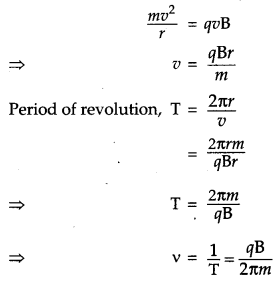Thus, frequency of revolution is independent of the energy of the particle. Yes, there is an upper limit on the energy acquired by the charged particle. The charged particle gains maximum speed when it moves in a path of radius equal to the radius of the dees.Question 29.
(a) Draw a ray diagram to show refraction of a ray of monochromatic light passing through a glass prism. Deduce the expression for the refractive index of glass in terms of angle of prism and angle of minimum deviation.
(b) Explain briefly how the phenomenon of total internal reflection is used in fibre optics. 
OR
(a) Obtain Lens Maker’s formula using the expressionHere the ray of light propagated from a rarer medium of refractive index (n1) to a denser medium of refractive index (n2) is incident on the convex side of spherical refracting surface of radius of curvature R.
(b)Draw a ray diagram to show the image formation by a concave mirror when the object is kept between its focus and the pole. Using this diagram, derive the magnification formula for the image formed.Each optical fiber consists of a core and cladding, refractive index of the material of the core is higher than that of cladding. When a signal, in the form of light, is directed into the optical fiber, at an angle greater than the critical angle, it undergoes repeated total internal reflections along the length of the fiber and comes out of it at the other end with almost negligible loss of intensity.Question 30.
(a) With the help of a labelled diagram, describe briefly the underlying principle and working of a step up transformer.
(b) Write any two sources of energy loss in a transformer.
(c) A step up transformer converts a low input voltage into a high output voltage. Does it violate law of conservation of energy ? Explain. 
OR
Derive an expression for the impedance of a series LCR circuit connected to an AC supply of variable frequency.
Plot a graph showing variation of current with the frequency of the applied voltage. Explain briefly how the phenomenon of resonance in the circuit can be used in the tuning mechanism of a radio or a TV set.
(a) Step – up transformer :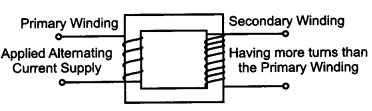Principle : It works on the principle of mutual induction. When alternating current is passed through a coil, an induced emf is set up in the neighbouring coil

Working: When an alternating voltage is applied to the primary, the resulting current produces an alternating magnetic flux which links the secondary and induces an emf in it. We consider an ideal transformer in which the primary has negligible resistance and all the flux in the core links with both primary and secondary windings. Let Φ be the flux in each hrm in the core at any time t due to current in the primary when a voltage Vp is applied to it. Then the induced emf or voltage Es in the secondary with Ns turns is(b) Sources of energy loss in transformer are : Joule’s loss in the resistance of winding’s, loss due to eddy currents.
(c) No. A step up transformer steps up the voltage while it steps down the current. So the input and output power remain same (provided there is no loss). Hence, there is no violation of the principle of energy conservation.
OR
Impedance (Z) in LCR circuit : The effective resistance offered by a series LCR circuit to the flow of current is called its impedance. It is denoted by Z.Suppose an inductance L, capacitance C and resistance R are connected in series to a source of alternating emf given by,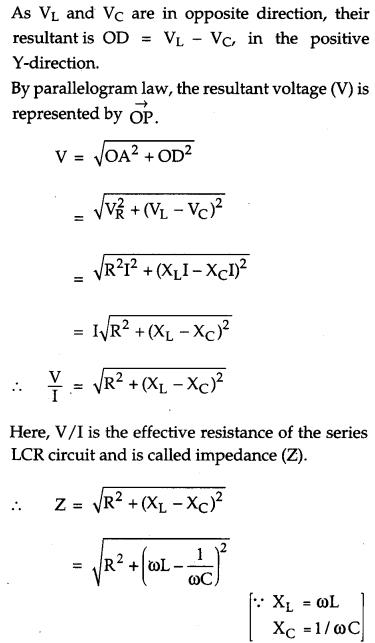The given graph shows the variation of current with the frequency of the applied voltage.The radio and TV receiver sets are the practical applications of series resonant circuits. Signals of several different frequencies are available in air. By turning the tuning knob of the radio set, we vary the frequency of the LC circuit till it matches the frequency of the desired signal.

### CBSE Previous Year Question Papers Class 12 Physics 2011 Delhi Set-II

Note : Except for the following questions, all the remaining questions have been asked in previous Set

Question 1.
The susceptibility of magnetic material is 1.9 x 10-5. Name the type of magnetic materials it represents. 
Paramagnetic substance because susceptibility of para-magnetic material is positive.

Question 2.
A plane electromagnetic wave travels in vacuum along X-direction. What can you say about the direction of electric and magnetic field vectors ? 
The electric and magnetic field vectors are in YZ-plane.

Question 3.
A magnet is quickly moved in the direction indicated by an arrow between coils C1 and C2 as shown in figure. What will be the direction of induced current in each coil as seen from the magnet ? Justify your answer.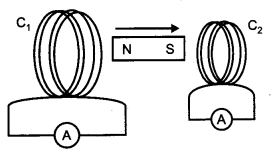For coil C1 : As the N-pole of the magnet is moving away from the coil C1, the end of the coil will behave as S-pole so as to oppose the motion of the magnet. Therefore, looking from the end, the current in the coil Q will be in clockwise direction.

For coil C2 : The end of the coil should behave as S-pole so as to repel the approaching magnet. Looking from the end, the direction of current in the coil of C2 will be in clockwise direction.

Question 4.
Figure shows two identical capacitors C and C2 each of 2 μF capacitance, connected to a . battery of 5 V. Initially switch ‘S’ is closed. After sometime ‘S’ is left open and dielectric slabs of dielectric constant k = 5 are inserted to fill completely the space between the plates of the two capacitors. How will the
(i) charge and
(ii) potential difference between the plates of the capacitors be affected after the slabs are inserted ?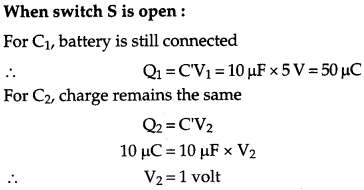Question 5.
How is forward biasing different from reverse biasing in a p-n junction diode ? 
Forward Biasing: The positive terminal of the external battery is connected to p-side and negative terminal of battery to n-side of p-n junction. The forward bias voltage oppose the potential barrier. Due to this, the potential barrier is reduced and hence the depletion becomes thin. The resistance of p-n region junction decreases.

Reverse biasing : The negative terminal of the external battery is connected to p-side and positive terminal of battery to n-side of p-n junction. The reverse bias voltage supports the potential barrier. Due to this, the potential barrier is increased. The resistance of p-n junction becomes high.

Question 6.
In the circuit shown, R1=4Ω, R2=R3=5Ω, R4=10Ω, and E = 6 V. Work out the equivalent resistance of the circuit and the current in each resistor.Question 7.
An electron and a photon each have a wavelength of 1.50 nm. Find:
(i) their momenta
(ii) the energy of the photon
(iii) the kinetic energy of the electron 
(i) For each electron or photon, momenta,### CBSE Previous Year Question Papers Class 12 Physics 2011 Delhi Set-III

Note : Except for the following questions, all the remaining questions have been asked in previous Set.

Question 1.
A plane electromagnetic wave travels in vacuum along Y-direction. What can you say about the direction of electric and magnetic field vectors ? 
For an electromagnetic wave propagating along Y-direction, the electric and magnetic field vectors vary sinusoidally along X-direction and Z-direction respectively.

Question 2.
The susceptibility of a magnetic material is -4.2 x 10-6. Name the type of magnetic materials it represents. 
Diamagnetic substance.

Question 3.
Explain how a depletion region is formed in a junction diode. 
With the formation of p-n junction, the holes from p-region diffuse into the n-region and electrons from n-region diffuse into the p-region and electron-hole pair combine and get annihilated. This in turn, produces potential barrier across the junction which opposes the further diffusion through the junction. Thus, a small region is formed which is depleted of free charge carriers and has only immobile ions. This region is called depletion region.

Question 4.
Figure shows two identical capacitors C1 and C2 each of 1.5 μF capacitance, connected to ? a battery of 2 V. Initially switch ‘S’ is closed. After sometime ‘S’ is left open and dielectric slabs of dielectric constant K=2 are inserted to fill completely the space between the plates of the two capacitors. How will the
(i) charge and
(ii) potential difference between the plates of the capacitors be affected after the slabs are inserted ?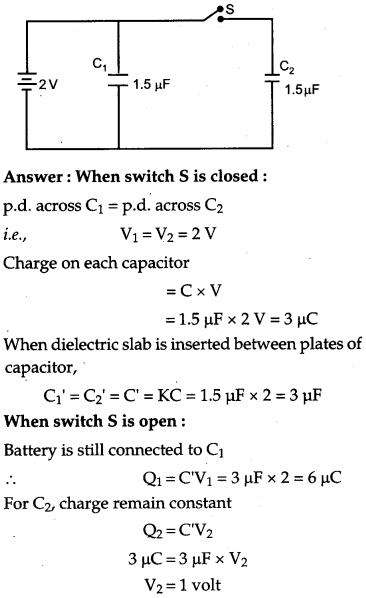Question 5.
Write the truth table for the logic circuit shown below and identify the logic operation by this circuit.Question 6.
Predict the polarity of the capacitor when the two magnets are quickly moved in the directions marked by arrows.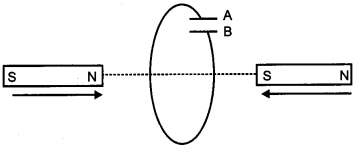In the situation shown, A will become positive with respect to B, as current induced is in clockwise direction.

Question 7.
In the circuit shown, R1=2 Ω, R2=R3=10 Ω, R4=20 Ω and E = 6 V. Work out the equivalent resistance of the circuit and the current in each resistor.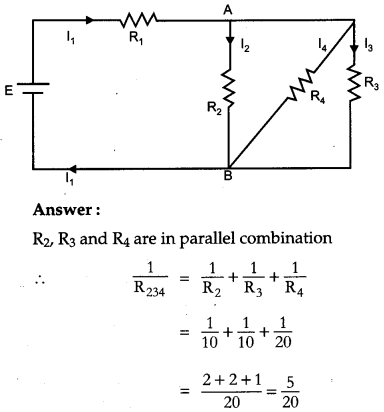Question 8.
An electron and a photon each have a wavelength of 2 nm. Find : 11
(i) their momenta
(ii) the energy of photon
(iii) the kinetic energy of the electron